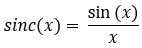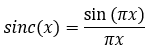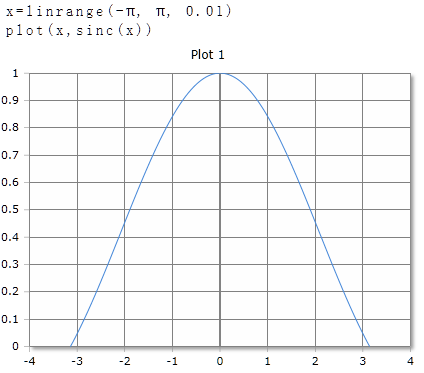# Sinc Function

Returns the cardinal sine of the argument (unnormalized sinc function)

## Information about the Cardinal Sine

The cardinal sine function or sinc function, denoted by sinc(x), has two slightly different definitions. The standard is not uniformly defined, especially in English-speaking countries sinc is used both for the normalized and for the non-normalized variant.

In mathematics, the unnormalized sinc function is defined for x ≠ 0 byIn digital signal processing and information theory, the normalized sinc function is commonly defined for x ≠ 0 by## Description

RedCrab supports the unnormalized $$Sinc$$ function. The function returns the cardinal sine of real numbers. The argument can be a single number or a data field. For data fields, the cardinal sine of each element is calculated and the results returned in a data field of equal size.

### Parameter

The angle is given as a real number in degrees (full circle = 360°) or radians (full circle = 2 · π). The unit of measurement is set in the toolbar with the DEG or RAD buttons. The Setting applies to the entire worksheet.

### Result

Sinc returns a result in the range of -1 to 1.

### Optional Parameter

Optionally, a second parameter with the keywords DEG or RAD can be specified to set the unit of measure for this function call. The specification of the parameter has priority over the global setting in the toolbar. For different functions, you can use different units of measurement regardless of the default setting in the toolbar.

### Syntax

Sinc (x)